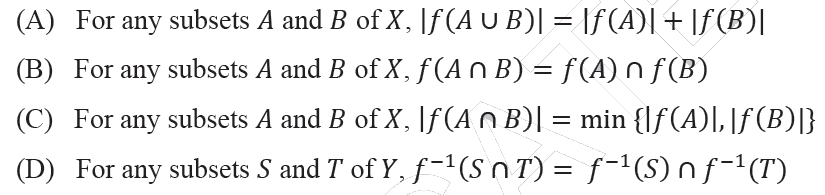# GATE | GATE-CS-2014-(Set-3) | Question 12

• Difficulty Level : Basic
• Last Updated : 28 Jun, 2021

Let X and Y be finite sets and f: X -> Y be a function. Which one of the following statements is TRUE?(A) A
(B) B
(C) C
(D) D

Explanation:

```Let x = {a, b, c} and y = {1, 2}
A Function f maps each element of x to 1 in y.
f(a)=1 , f(b)=1 , f(c) =1
A = {a, b} B = {b, c}
----------------------------------------------
A ]
| f(A u B) | = |f({a, b, c})| = 3
| f(A)|+|f(B)| = 2 + 2 = 4 , LHS != RHS.
----------------------------------------------
B ]
f(A ∩ B) = f({b}) = { 1 }
f(A) ∩ f(B) = {1, 1} ∩ {1, 1} = {1, 1}
LHS != RHS
-----------------------------------------------
C ]
|f(A ∩ B)| = |f({b})| = |{ 1 }| = 1
min{|f(A)|,|f(B)|} = min(2,2) = 2
LHS != RHS
-----------------------------------------------
D ] In a function a value can be mapped only to one value.```

Attention reader! Don’t stop learning now.  Practice GATE exam well before the actual exam with the subject-wise and overall quizzes available in GATE Test Series Course.

Learn all GATE CS concepts with Free Live Classes on our youtube channel.

My Personal Notes arrow_drop_up